Vibroengineering PROCEDIA

Published: 25 March 2021

# Analysis of influence of bridge deck damage on first order natural frequency of simply supported T-beam bridge

Shuichang Li1
1Guangxi Nantian Expressway Co., Ltd, Nanning, China
Views 133

#### Abstract

As a simply-supported T beam of the girder grid system, the damage of the bridge deck will inevitably change the overall stiffness of the bridge, and then affect the natural dynamic characteristics of the bridge. The variation of the first-order natural frequency of the bridge before and after the damage of the T beam flange was analyzed by using the finite element analysis method, and the influence law of the T beam flange damage on the natural frequency of the simply supported T beam bridge was obtained. The analysis results showed that the damage of T beam flange will bring about a significant change in the first-order natural frequency. Taking the natural frequency test of multi-span simply supported beams in practical engineering as an example, the first order natural frequency of the undamaged span and the damaged span of the bridge deck were tested respectively, and the influence of the damage on the first order natural frequency was analyzed. Results showed that the first order natural frequency decreases by 15.63 % under the condition that the single T beam is completely damaged in the mid-span position. Therefore, the damage of T bridge can be preliminarily judged by the change of the natural frequency of simply supported beam bridge in practical engineering.

## 1. Introduction

With the extension of operation time, the service performance of the bridge will decline in the actual operation process, resulting in different degrees of disease . Heavy vehicle traffic is very likely to occur, especially in some Chinese national roads, which will accelerate the attenuation of bridge performance and easily lead to damage and other diseases [2, 3].

Reinforced concrete simply supported T beam has been widely used in bridge construction since 1990s. A main feature of this bridge type is the use of beam lattice system, which is formed by multi-slice T beam assembly. During the assembly process, T beam is connected by the wet connection of the wing plate, which plays the role of bearing the bridge deck. T beam flange is also the most vulnerable position under the reciprocating action of heavy vehicle, especially near the middle span of bridge deck center line. This kind of damage will inevitably cause the change of the dynamic and static characteristics of the bridge [4, 5]. It is a hot topic for scholars in recent years to identify such damage by the change of dynamic characteristics [6, 7]. Some literatures have shown that the damage of bridge will cause the change of the natural dynamic characteristics of bridge, such as natural frequency, mode, and so on . Therefore, it is of great significance to study the influence degree of bridge damage on the change of dynamic characteristics, and to identify the bridge damage by using the measured dynamic characteristics.

Taking the reinforced concrete simply supported T beam bridge as the object, this paper studied and analyzes the influence of T beam flange damage (bridge deck damage) on the natural frequency of the bridge. By using the finite element method, the calculation models of damaged and undamaged bridge deck were established respectively, and the natural frequency variation calculated by the theory was compared. Also, based on an actual multi span simply supported beam bridge, the measured natural frequencies of undamaged span and damaged span were compared and tested. Finally, the effects of deck damage on the first order natural frequencies of simply supported T bridges were analyzed by theoretical and experimental methods. As a result, it provides a basis for identifying the damage of bridges by using the variation of the natural frequency of simply supported T bridges in engineering.

## 2. Theoretical calculation method for the first order natural frequency of simple supported beam

The simply supported beam bridge can be simplified according to the mechanics as the dynamic analysis.

The vibration equilibrium equation of simply supported beam can be formed by the moment balance equation and force balance equation:

1
$EI\frac{{\partial }^{4}y\left(x,t\right)}{\partial {x}^{4}}+m\frac{{\partial }^{2}y\left(x,t\right)}{\partial {t}^{2}}=0.$

The formula of natural frequency theory of simply supported beam is obtained by solving Eq. (1):

2

where ${\omega }_{n}$ is the n order natural circular frequency of simply supported beam, $m$ is the mass of unit length of simply supported beam, and $L$ is the calculated span of simply supported beam. $EI$ is the bending stiffness of simply supported beam section.

From Eq. (2), it can be seen that in the case of constant span of bridge, the change of bending stiffness $EI$ of simply supported beam section will inevitably bring about the change of circular frequency ${\omega }_{n}$.

For the T beam bridge, the damage of the wing plate of the T section will lead to the decrease of the moment of inertia of the section $I$, and then the bending stiffness of the section $EI$ will be reduced. Hence, according to Eq. (2), the natural frequency of the T beam will be reduced due to the breakage of the wing plate of the T section.

## 3. Finite element analysis of the effect of flange breakage on first order natural frequency

### 3.1. Establishment of finite element model

Taking a simply supported T beam bridge with a span of 22 m as an example, a finite element model was established to analyze the effect of bridge panel damage on the first order natural frequency. Bridge transverse is composed of 7 pieces of T beam, the width of middle beam and side beam is the same, and the steel plate welding connection is used between T beams. The web bottom width of each T beam is 0.36 m, the web height is 1.30 m, and the cantilever section width of one side of the flange plate is 0.70 m (see Fig. 1 for the section size of the T beam). Beam concrete material is C40 concrete. A finite element model of the bridge was established to simulate the sound condition and damage condition of the deck of middle and 3# and 4#T span are all damaged. The finite element model is shown in Fig. 2.

### 3.2. Calculation results and comparison of natural frequencies before and after damage

From the finite element calculation result, the first mode of simply supported beam was obtained as shown in Fig. 3. From this, the calculation results of natural frequency before and after damage were obtained, as shown in Table 1.

Fig. 1Cross section of T beam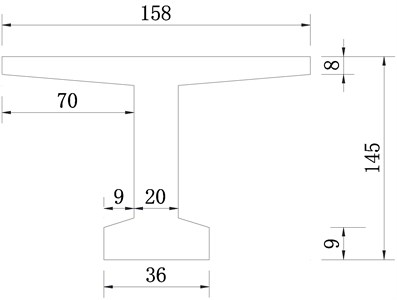Fig. 2Finite element model of simply supported T beam bridge based on beam grid method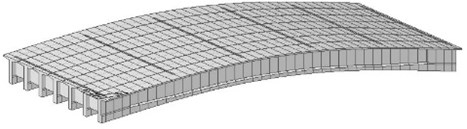Table 1Comparison of natural frequencies before and after damage

 Conditions First order natural frequency (Hz) Frequency reduction percentage Undamaged condition 5.285 – Damaged condition 4.492 –15 %

Fig. 3First order natural mode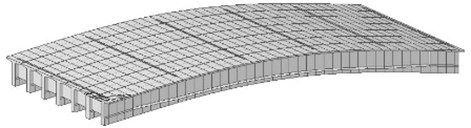It can be obtained from Table 1 that the first order natural frequency is obviously reduced under the condition of no damage, and the reduction ratio reaches 15 %.

## 4. Real bridge test on the effect of wing plate breakage on first order natural frequency

### 4.1. Bridge profile

A bridge is located in Guangxi Hepu County Nanning to Beihai second class highway 325 line, built in 1990.The total length of the bridge is 330.94 m, the total width of the bridge deck is 12.50 m, the superstructure bridge hole is arranged as 8.50 m (cast-in-place reinforced concrete slab beam) 14×22.20 m (precast reinforced concrete simply supported T slab beam) 8.50 (cast-in-place reinforced concrete slab beam), and the T beam support is plate rubber bearing. There are 14 reinforced concrete simply supported T beams with span of 22.2 m. The bridge cross section diagram is shown in Fig. 4.

### 4.2. Damage to bridges

Due to the long construction time of the bridge, the service performance has been decayed, and the heavy vehicle traffic volume on the national highway causes the bridge deck (wing plate) of multi span T-beam to be broken in varying degrees. Among them, 6# span T beam flange is damaged most seriously, in the middle of the span between the two transverse partitions, two pieces of T beam between the flange all broken, as shown in Fig. 5. And the bridge 14# span and 15# are undamaged spans.

Fig. 4Cross-sectional view (cm)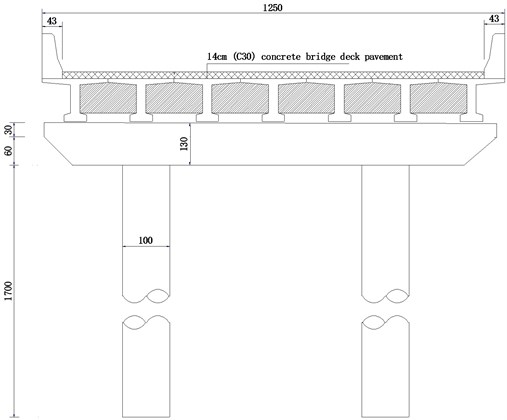Fig. 5Serious breakage of T beam flange in middle span of 6# span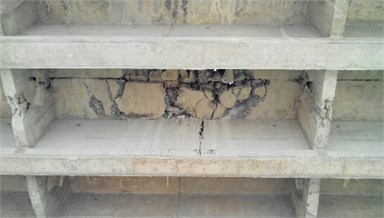### 4.3. Testing and comparative analysis of first order natural frequency

According to the above analysis, the natural frequency of the 6# span T beam flange will decrease after damage and its natural frequency will be significantly smaller compared with the undamaged 14# span and 15# span. For this reason, the natural frequencies of damaged and undamaged span were identified by pulsating test. By using the DH610V acceleration picker tested by Donghua, the vibration acceleration time history curves of 6# span, 14# span and 15# span were collected. The typical acceleration time history curves are shown in Fig. 6.

Using the acceleration versus time data to analyze the spectrum and the vibration spectra of 6# span, 14# span and 15# span were obtained which are shown in Figs. 7 and 8. Then identify the first order natural frequencies of each span, the comparison are shown in Table 2.

It can be seen from Table 2 that the first order natural frequency of 6# span with serious deck damage is significantly lower than that of 14# and 15# spans with undamaged deck, and the reduction percentage is 15.63 %, which is consistent with the theoretical calculation results in Table 1. Hence, the change of the natural frequency of simply supported T beam bridge can reflect the damage of the bridge in engineering.

Fig. 6Time history curve of acceleration in midspan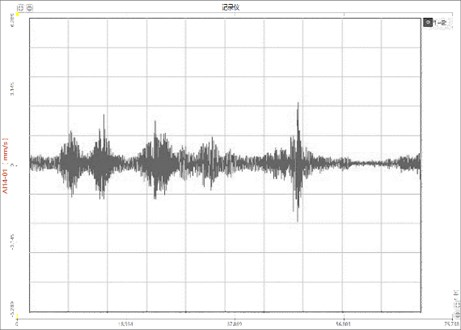Fig. 7Spectrogram of 14# span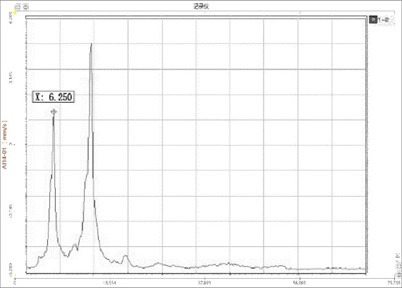Fig. 8Spectrogram of 6# span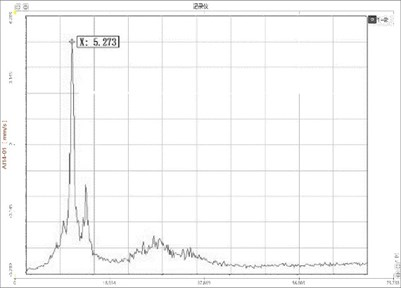Table 2Comparison of first order natural frequency

 Spans First order natural frequency Frequency reduction percentage Calculated value (Hz) Measured value (Hz) Measured value / Calculated value 6# span 4.492 5.273 1.174 –15.63 % 14# span 5.285 6.250 1.183 0 % 15# span 5.285 6.250 1.183 0 %

## 5. Conclusions

In this paper, the change of the first order natural frequency of the simply supported T beam bridge before and after the damage of the bridge deck were analyzed with finite element analysis method, and the influence law of the damage of the T beam flange on the natural frequency of the simply supported T beam bridge was obtained. By using the method of engineering contrast test, the first order natural frequencies of damaged span and undamaged span of T beam flange plate under the condition of the same span and cross-section were tested. The following conclusions were drawn:

1) For T beam bridge, the damage of T shaped section flange will lead to the reduction of the cross-section moment of inertia, which will lead to the reduction of the bending stiffness of the section, and finally cause the obvious reduction of the natural frequency of the T beam.

2) The first order natural frequency of simply supported T beam bridge is obviously reduced when the airfoil between two transverse partitions and two beams is completely broken at the middle of the span. The contrast test results showed that the reduction percentage reached 15.63 %.

3) The change of the natural frequency of simply supported T beam bridge can reflect the damage of the bridge in engineering.